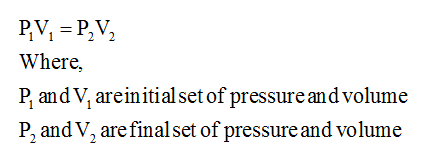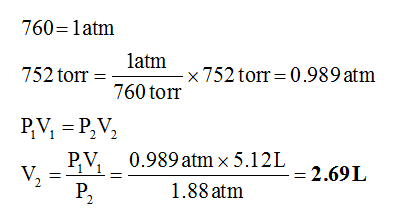# A fixed quantity of gas at 21 °C exhibits a pressure of 752torr and occupies a volume of 5.12 L. (a) Calculate the volumethe gas will occupy if the pressure is increased to 1.88 atm while the temperature is held constant. (b) Calculate thevolume the gas will occupy if the temperature is increased to175 °C while the pressure is held constant.

Question
8 views

A fixed quantity of gas at 21 °C exhibits a pressure of 752
torr and occupies a volume of 5.12 L. (a) Calculate the volume
the gas will occupy if the pressure is increased to 1.88 atm while the temperature is held constant. (b) Calculate the
volume the gas will occupy if the temperature is increased to
175 °C while the pressure is held constant.

check_circle

Step 1

(a) In this part, both the volume and pressure are variable but temperature is held constant. So Boyle’s law has to be used in order to solve this. The mathematical form of Boyle’s law is given below.Step 2

By substituting the given data in the above expression, the value of asked volume can be calculated.Therefore, the volume the gas will occupy is 2.69 L.

...

### Want to see the full answer?

See Solution

#### Want to see this answer and more?

Solutions are written by subject experts who are available 24/7. Questions are typically answered within 1 hour.*

See Solution
*Response times may vary by subject and question.
Tagged in